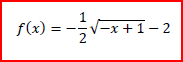Clutch Prep is now a part of Pearson
Ch.2: Pre-Calc (Part 2)WorksheetSee all chapters

# Transformations of Functions

See all sections
Sections
Evaluating Functions
Composition of Functions
Transformations of Functions
Symmetry
Piece-Wise Functions

Concept #1: Part 1

Concept #2: Intro

Practice: Graph the following transformation.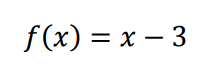Practice: Graph the following transformation.Practice: Graph the following transformation.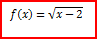Practice: Graph the following transformation.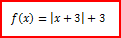Concept #3: Part 2

Concept #4: Intro

Practice: Graph the following transformation.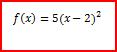Practice: Graph the following transformation.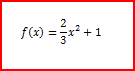Practice: Graph the following transformation.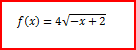Practice: Graph the following transformation.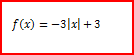Practice: Graph the following transformation.